## Example Questions

### Example Question #1 : Diameter

The perimeter of a circle is 36 π.  What is the diameter of the circle?

72

6

3

18

36

36

Explanation:

The perimeter of a circle = 2 πr = πd

Therefore d = 36

### Example Question #1 : How To Find The Length Of The Diameter

Two legs of a right triangle measure 3 and 4, respectively. What is the area of the circle that circumscribes the triangle?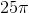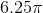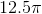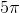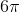Explanation:

For the circle to contain all 3 vertices, the hypotenuse must be the diameter of the circle. The hypotenuse, and therefore the diameter, is 5, since this must be a 3-4-5 right triangle.

The equation for the area of a circle is A = πr2.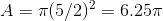### Example Question #1 : How To Find The Length Of The Diameter

If a circle has an area of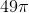, what is the diameter of the circle?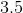Explanation:

1. Use the area to find the radius: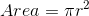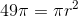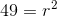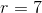2. Use the radius to find the diameter: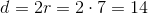### Example Question #2 : How To Find The Length Of The Diameter

What is the diameter of a semi-circle that has an area of?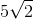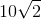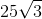Explanation:

To begin, be very careful to note that the question asks about a semi-circle—not a complete circle! This means that a complete circle composed out of two of these semi-circles would have an area of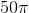. Now, from this, we can use our area formula, which is:For our data, this is: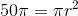Solving for, we get: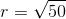This can be simplified to: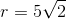The diameter is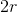, which is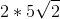or.

### Example Question #3 : How To Find The Length Of The Diameter

A circle has an area of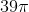.  What is the diameter of the circle?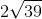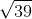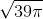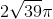Explanation:

The equation for the area of a circle is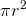, which in this case equals.  Therefore,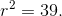The only thing squared that equals an integer (which is not a perfect root) is that number under a square root.  Therefore,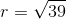.  Since diameter is twice the radius,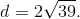### Example Question #1 : How To Find The Length Of The Diameter

Find the diameter given the radius is.Explanation:

Diameter is simply twice the radius. Therefore,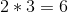.

### Example Question #2 : How To Find The Length Of The Diameter

Find the length of the diameter of a circle given the area is.Explanation:

To solve, simply use the formula for the area of a circle, solve for r, and multiply by 2 to get the diameter. Thus,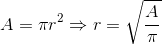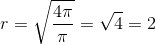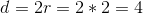### Example Question #6 : How To Find The Length Of The Diameter

In a group of students, it was decided that a pizza would be divided according to its crust size. Every student wanted 3 inches of crust (measured from the outermost point of the pizza). If the pizza in question had a diameter of 14 inches, what percentage of the pizza was wasted by this manner of cutting the pizza? Round to the nearest hundredth.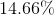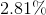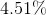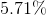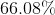Explanation:

What we are looking at is a way of dividing the pizza according to arc lengths of the crust. Thus, we need to know the total circumference first. Since the diameter is, we know that the circumference is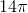. Now, we want to ask how many ways we can divide up the pizza into pieces ofinch crust. This is: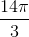or approximately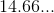pieces.

What you need to do is take this amount and subtract off. This is the amount of crust that is wasted. You can then merely divide it by the original amount of divisions: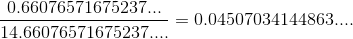(You do not need to work in exact area or length. These relative values work fine.)

This is aboutof the pizza that is wasted.

### Example Question #7 : How To Find The Length Of The Diameter

A circle has an area of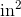. What is its diameter?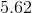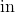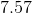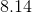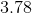Explanation:

To solve a question like this, first remember that the area of a circle is defined as: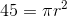To solve for, first divide both sides by. Then take the square root of both sides. Thus you get: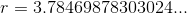The diameter of the circle is just double that: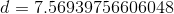Rounding to the nearest hundredth, you get.